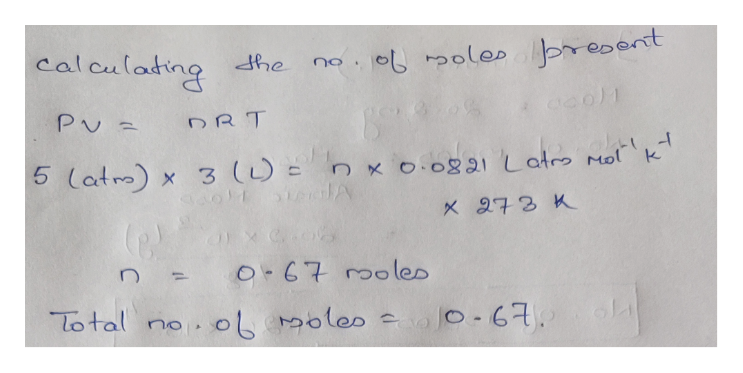# Suppose that Daniel has a 3.003.00 L bottle that contains a mixture of O2O2, N2N2, and CO2CO2 under a total pressure of 5.005.00 atm. He knows that the mixture contains 0.270.27 mol N2N2 and that the partial pressure of CO2CO2 is 0.3500.350 atm. If the temperature is 273273 K, what is the partial pressure of O2O2?

Question
103 views

Suppose that Daniel has a 3.003.00 L bottle that contains a mixture of O2O2, N2N2, and CO2CO2 under a total pressure of 5.005.00 atm. He knows that the mixture contains 0.270.27 mol N2N2 and that the partial pressure of CO2CO2 is 0.3500.350 atm. If the temperature is 273273 K, what is the partial pressure of O2O2?

check_circle

Step 1

To calculate the partial pressure of oxygen, first it is required to calculate the total number of moles present, which can be done by using the ideal gas equation,help_outlineImage Transcriptionclosecalaulattng olespreoent dhe no e0)1 PU DRT 5 latro) 3 (L n K O.0891 Latro Mol k STCHIA Xelo8 O-67 00 les Total no 0L SLa Moles o - 6 fullscreen
Step 2

Now calculating the mole fraction of nitrogen gas,

Step 3

Now the partial pressuer of nitro...

### Want to see the full answer?

See Solution

#### Want to see this answer and more?

Solutions are written by subject experts who are available 24/7. Questions are typically answered within 1 hour.*

See Solution
*Response times may vary by subject and question.
Tagged in

### Chemistry